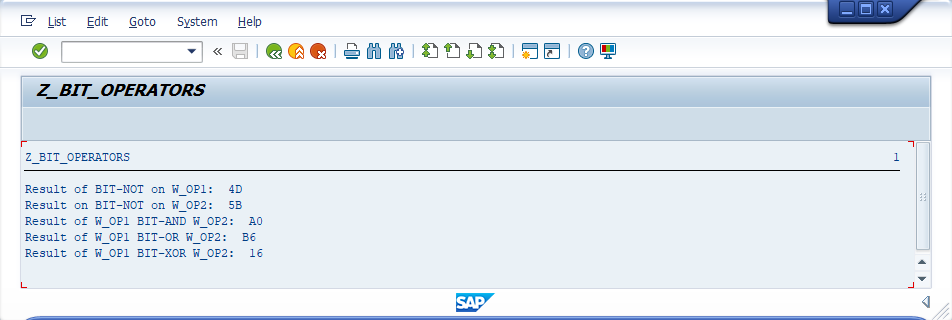###### Summary -

In this topic, we described about the below sections -

The bit operators perform bit operations on two or more byte-like operands. ABAP supports a series of bit logical operators. Bit operators used to process Boolean algebraic expressions.

Bit expression formulates a binary calculation. Bit expressions are combined by using braces to formulate complex bit expressions.

Bit operators work on the individual bits of the operands. The bit operators BIT-AND, BIT-OR and BIT-XOR joins two adjacent operands.

The bit operator BIT-NOT negates a byte-like operand. BIT-NOT can be stated one or more times before an operand to negate the value of the operand. If BIT-NOT is specified an even number of times, the operand value remains unchanged. If BIT-NOT is specified an odd number of times, the operand value get changed.

Below table shows list of the arithmetic operators -

OperatorDescriptionPriority
BIT-NOTUnary operator performs NOT operation on bit by bit. BIT-NOT flips the bits in hexadecimal number to opposite value.1
BIT-ANDBinary operator performs AND operation on bit by bit2
BIT-XORBinary operator performs Exclusive OR operation on bit by bit3
BIT-ORBinary operator performs OR operation on bit by bit4

Below table describes how the BIT-AND, BIT-OR, BIT-XOR and BIT-NOT operations worked on individual bits.

Operand1Operand2Operand1 BIT-AND Operand2Operand1 BIT-OR Operand2Operand1 BIT-XOR Operand2BIT-NOT Operand1BIT-NOT Operand2
0000011
0101110
1001101
1111000

#### Example -

Below example shows how the bitwise operations coded in the program.

#### Code -

``````*&---------------------------------------------------------------------*
*& Report  Z_BIT_OPERATORS
*&---------------------------------------------------------------------*
*& Written by TutorialsCampus
*&---------------------------------------------------------------------*

REPORT  Z_BIT_OPERATORS.

* Declaring variables
DATA: W_OP1 TYPE X VALUE 'B2',
W_OP2 TYPE X VALUE 'A4',
W_NOT TYPE X,
W_AND TYPE X,
W_OR  TYPE X,
W_XOR TYPE X.

* Applying BIT-NOT on W_OP1 and produces the result W_NOT.
W_NOT = BIT-NOT W_OP1.
WRITE: 'Result of BIT-NOT on W_OP1: ',W_NOT.

* Applying BIT-NOT on W_OP2 and produces the result W_NOT.
W_NOT = BIT-NOT W_OP2.
WRITE: /'Result on BIT-NOT on W_OP2: ',W_NOT.

* Applying BIT-AND on W_OP1, W_OP2 and produces the result W_AND.
W_AND = W_OP1 BIT-AND W_OP2.
WRITE: /'Result of W_OP1 BIT-AND W_OP2: ',W_AND.

* Applying BIT-OR on W_OP1, W_OP2 and produces the result W_OR.
W_OR = W_OP1 BIT-OR W_OP2.
WRITE: /'Result of W_OP1 BIT-OR W_OP2: ',W_OR.

* Applying BIT-XOR on W_OP1, W_OP2 and produces the result W_XOR.
W_XOR = W_OP1 BIT-XOR W_OP2.
WRITE: /'Result of W_OP1 BIT-XOR W_OP2: ',W_XOR.
``````

#### Output -#### Explaining Example -

In the above example, each and every statement is preceeded with a comment to explain about the statement. Go through them to get clear understanding of example code.

W_NOT = BIT-NOT W_OP1, applies BIT-NOT on W_OP1 and produces the result W_NOT. W_NOT = BIT-NOT W_OP2, applies BIT-NOT on W_OP2 and produces the result W_NOT.

W_AND = W_OP1 BIT-AND W_OP2, applies BIT-AND on W_OP1, W_OP2 and produces the result W_AND. W_OR = W_OP1 BIT-OR W_OP2, applies BIT-OR on W_OP1, W_OP2 and produces the result W_OR. W_XOR = W_OP1 BIT-XOR W_OP2, applies BIT-XOR on W_OP1, W_OP2 and produces the result W_XOR.